This article was published as a part of the Data Science Blogathon

## Introduction

Linear Regression, a supervised technique is one of the simplest Machine Learning algorithms. It is a linear approach to modeling the relationship between a scalar response and one or more explanatory variables.

Therefore it becomes necessary for every aspiring Data Scientist and Machine Learning Engineer to have a good knowledge of the Linear Regression Algorithm.

In this article, we will discuss the most important questions on the Linear Regression Algorithm which is helpful to get you a clear understanding of the Algorithm, and also for Data Science Interviews, which covers its very fundamental level to complex concepts.

## 1. What is Linear Regression Algorithm?

In simple terms: It is a method of finding the best straight line fitting to the given dataset, i.e. tries to find the best linear relationship between the independent and dependent variables.

In technical terms: It is a supervised machine learning algorithm that finds the best linear-fit relationship on the given dataset, between independent and dependent variables. It is mostly done with the help of the Sum of Squared Residuals Method, known as the Ordinary least squares (OLS) method.## 2. How do you interpret a linear regression model?

As we know that the linear regression model is of the form: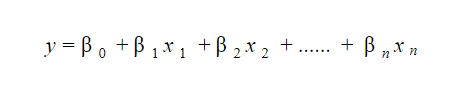The significance of the linear regression model lies in the fact that we can easily interpret and understand the marginal changes in the independent variables(predictors) and observed their consequences on the dependent variable(response).

Therefore, a linear regression model is quite easy to interpret.

For Example, if we increase the value of x1 increases by 1 unit, keeping other variables constant, then the total increase in the value of y will be βi and the intercept term (β0) is the response when all the predictor’s terms are set to zero or not considered.

## 3. What are the basic assumptions of the Linear Regression Algorithm?

The basic assumptions of the Linear regression algorithm are as follows:

• Linearity: The relationship between the features and target.
• Homoscedasticity: The error term has a constant variance.
• Multicollinearity: There is no multicollinearity between the features.
• Independence: Observations are independent of each other.
• Normality: The error(residuals) follows a normal distribution.

Now, let’s break these assumptions into different categories:

### Assumptions about the form of the model:

It is assumed that there exists a linear relationship between the dependent and the independent variables. Sometimes, this assumption is known as the ‘linearity assumption’.

• Normality assumption: The error terms, ε(i), are normally distributed.
• Zero mean assumption: The residuals have a mean value of zero.
• Constant variance assumption: The residual terms have the same (but unknown) value of variance, σ2. This assumption is also called the assumption of homogeneity or homoscedasticity.
• Independent error assumption: The residual terms are independent of each other, i.e. their pair-wise covariance value is zero.

• The independent variables are measured without error.
• There does not exist a linear dependency between the independent variables, i.e. there is no multicollinearity in the data.

## 4. Explain the difference between Correlation and Regression.

Correlation: It measures the strength or degree of relationship between two variables. It doesn’t capture causality. It is visualized by a single point.Regression: It measures how one variable affects another variable. Regression is all about model fitting. It tries to capture the causality and describes the cause and the effect. It is visualized by a regression line.

## 5. Explain the Gradient Descent algorithm with respect to linear regression.

Gradient descent is a first-order optimization algorithm. In linear regression, this algorithm is used to optimize the cost function to find the values of the βs (estimators) corresponding to the optimized value of the cost function.The working of Gradient descent is similar to a ball that rolls down a graph (ignoring the inertia). In that case, the ball moves along the direction of the maximum gradient and comes to rest at the flat surface i.e, corresponds to minima.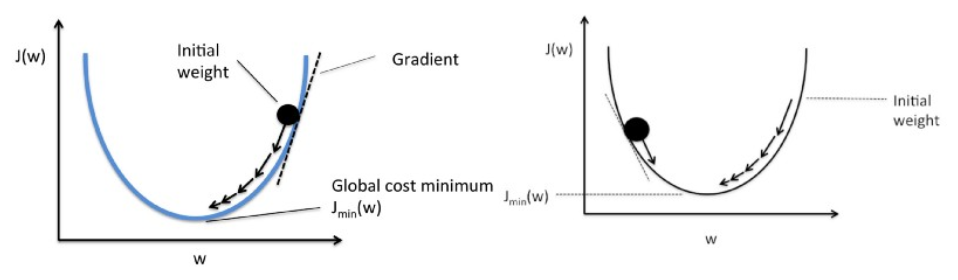### Now, let’s understand it mathematically:

Mathematically, the main objective of the gradient descent for linear regression is to find the solution of the following expression,

ArgMin J(θ0, θ1), where J(θ0, θ1) represents the cost function of the linear regression. It is given by :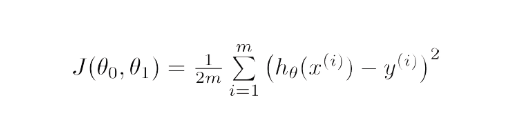Here, h is the linear hypothesis model, defined as h=θ0 + θ1x,

y is the target column or output, and m is the number of data points in the training set.

### Steps of Gradient Descent Algorithm:

Step-1: Gradient Descent starts with a random solution,

Step-2: Based on the direction of the gradient, the solution is updated to the new value where the cost function has a lower value.

The updated value for the parameter is given by the formulae:

Repeat until convergence(upto minimum loss function)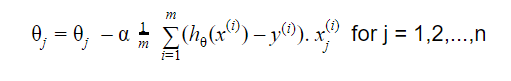## 6. Justify the cases where the linear regression algorithm is suitable for a given dataset.

Generally, a Scatter plot is used to see if linear regression is suitable for any given data. So, we can go for a linear model if the relationship looks somewhat linear. Plotting the scatter plots is easy in the case of simple or univariate linear regression.But if we have more than one independent variable i.e, the case of multivariate linear regression, then two-dimensional pairwise scatter plots, rotating plots, and dynamic graphs can be plotted to find the suitableness.

On the contrary, to make the relationship linear we have to apply some transformations.

## 7. List down some of the metrics used to evaluate a Regression Model.

Mainly, there are five metrics that are commonly used to evaluate the regression models:

• Mean Absolute Error(MAE)
• Mean Squared Error(MSE)
• Root Mean Squared Error(RMSE)
• R-Squared(Coefficient of Determination)

## 8. For a linear regression model, how do we interpret a Q-Q plot?

The Q-Q plot represents a graphical plotting of the quantiles of two distributions with respect to each other. In simple words, we plot quantiles against quantiles in the Q-Q plot which is used to check the normality of errors.Whenever we interpret a Q-Q plot, we should concentrate on the ‘y = x’ line, which corresponds to a normal distribution. Sometimes, this line is also known as the 45-degree line in statistics.

It implies that each of the distributions has the same quantiles. In case you witness a deviation from this line, one of the distributions could be skewed when compared to the other i.e, normal distribution.

## 9. In linear regression, what is the value of the sum of the residuals for a given dataset? Explain with proper justification.

The sum of the residuals in a linear regression model is 0 since it assumes that the errors (residuals) are normally distributed with an expected value or mean equal to 0, i.e.Y = βT X + ε

Here, Y is the dependent variable or the target column, and β is the vector of the estimates of the regression coefficient,

X is the feature matrix containing all the features as the columns, ε is the residual term such that ε ~ N(0, σ2).

Moreover, the sum of all the residuals is calculated as the expected value of the residuals times the total number of observations in our dataset. Since the expectation of residuals is 0, therefore the sum of all the residual terms is zero.

Note: N(μ, σ2) denotes the standard notation for a normal distribution having mean μ and standard deviation σ2.
<

## 10. What are RMSE and MSE? How to calculate it?

RMSE and MSE are the two of the most common measures of accuracy for linear regression.

MSE (Mean Squared Error) is defined as the average of all the squared errors(residuals) for all data points. In simple words, we can say it is an average of squared differences between predicted and actual values.

RMSE (Root Mean Squared Error) is the square root of the average of squared differences between predicted and actual values.

RMSE stands for Root mean square error, which represented by the formulae: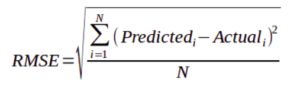MSE stands for Mean square error, which represented by the formulae: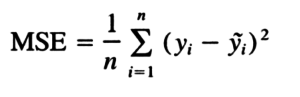### Conclusion:

Increment in RMSE is larger than MAE as the test sample size increases. In general, as the variance of error magnitudes increase, MAE remains steady but RMSE increases.

## 11. What is OLS?

OLS stands for Ordinary Least Squares. The main objective of the linear regression algorithm is to find coefficients or estimates by minimizing the error term i.e, the sum of squared errors. This process is known as OLS.This method finds the best fit line, known as regression line by minimizing the sum of square differences between the observed and predicted values.

## 12. What are MAE and MAPE?

MAE stands for Mean Absolute Error, which is defined as the average of absolute or positive errors of all values. In simple words, we can say MAE is an average of absolute or positive differences between predicted values and the actual values.MAPE stands for Mean Absolute Percent Error, which calculates the average absolute error in percentage terms. In simple words, It can be understood as the percentage average of absolute or positive errors.## 13. Why do we square the residuals instead of using modulus?

This question can be understood that why one should prefer the absolute error instead of the squared error.1. In fact, the absolute error is often closer to what we want when making predictions from our model. But, if we want to penalize those predictions that are contributing to the maximum value of error.

2. Moreover in mathematical terms, the squared function is differentiable everywhere, while the absolute error is not differentiable at all the points in its domain(its derivative is undefined at 0). This makes the squared error more preferable to the techniques of mathematical optimization. To optimize the squared error, we can compute the derivative and set its expression equal to 0, and solve. But to optimize the absolute error, we require more complex techniques having more computations.

3. Actually, we use the Root Mean Squared Error instead of Mean squared error so that the unit of RMSE and the dependent variable are equal and results are interpretable.

## 14. List down the techniques that are adopted to find the parameters of the linear regression line which best fits the model.

There are mainly two methods used for linear regression:1. Ordinary Least Squares(Statistics domain):

To implement this in Scikit-learn we have to use the LinearRegression() class.

To implement this in Scikit-learn we have to use the SGDRegressor() class.

## 15. Which evaluation metric should you prefer to use for a dataset having a lot of outliers in it?

Mean Absolute Error(MAE) is preferred when we have too many outliers present in the dataset because MAE is robust to outliers whereas MSE and RMSE are very susceptible to outliers and these start penalizing the outliers by squaring the error terms, commonly known as residuals.

## 16. Explain the normal form equation of the linear regression.

The normal equation for linear regression is :β=(XTX)-1XTY

This is also known as the closed-form solution for a linear regression model.

where,

Y=βTX is the equation that represents the model for the linear regression,

Y is the dependent variable or target column,

β is the vector of the estimates of the regression coefficient, which is arrived at using the normal equation,

X is the feature matrix that contains all the features in the form of columns. The thing to note down here is that the first column in the X matrix consists of all 1s, to incorporate the offset value for the regression line.

## 17. When should it be preferred to the Gradient Descent method instead of the Normal Equation in Linear Regression Algorithm?

To answer the given question, let’s first understand the difference between the Normal equation and Gradient descent method for linear regression:

• Needs hyper-parameter tuning for alpha (learning parameter).
• It is an iterative process.
• Time complexity- O(kn2)
• Preferred when n is extremely large.

### Normal Equation:

• No such need for any hyperparameter.
• It is a non-iterative process.
• Time complexity- O(n3) due to evaluation of XTX.
• Becomes quite slow for large values of n.

where,

‘k’ represents the maximum number of iterations used for the gradient descent algorithm, and

‘n’ is the total number of observations present in the training dataset.

Clearly, if we have large training data, a normal equation is not preferred for use due to very high time complexity but for small values of ‘n’, the normal equation is faster than gradient descent.

## 18. What are R-squared and Adjusted R-squared?

R-square (R2), also known as the coefficient of determination measures the proportion of the variation in your dependent variable (Y) explained by your independent variables (X) for a linear regression model.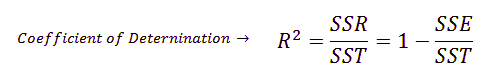The main problem with the R-squared is that it will always remain the same or increases as we are adding more independent variables. Therefore, to overcome this problem, an Adjusted-R2 square comes into the picture by penalizing those adding independent variables that do not improve your existing model.

## 19. What are the flaws in R-squared?

There are two major flaws of R-squared:Problem- 1: As we are adding more and more predictors, R² always increases irrespective of the impact of the predictor on the model. As R² always increases and never decreases, it can always appear to be a better fit with the more independent variables(predictors) we add to the model. This can be completely misleading.

Problem- 2: Similarly, if our model has too many independent variables and too many high-order polynomials, we can also face the problem of over-fitting the data. Whenever the data is over-fitted, it can lead to a misleadingly high R² value which eventually can lead to misleading predictions.

## 20. What is Multicollinearity?

It is a phenomenon where two or more independent variables(predictors) are highly correlated with each other i.e. one variable can be linearly predicted with the help of other variables. It determines the inter-correlations and inter-association among independent variables. Sometimes, multicollinearity can also be known as collinearity.### Reasons for Multicollinearity:

• Inaccurate use of dummy variables.
• Due to a variable that can be computed from the other variable in the dataset.

### Impacts of Multicollinearity:

• Impacts regression coefficients i.e, coefficients become indeterminate.
• Causes high standard errors.

### Detecting Multicollinearity:

• By using the correlation coefficient.
• With the help of Variance inflation factor (VIF), and Eigenvalues.

## 21. What is Heteroscedasticity? How to detect it?

It refers to the situation where the variations in a particular independent variable are unequal across the range of values of a second variable that tries to predict it.### Detect Heteroscedasticity:

To detect heteroscedasticity, we can use graphs or statistical tests such as the Breush-Pagan test and NCV test, etc.

## 22. What are the disadvantages of the linear regression Algorithm?

The main disadvantages of linear regression are as follows:

• Assumption of linearity: It assumes that there exists a linear relationship between the independent variables(input) and dependent variables (output), therefore we are not able to fit the complex problems with the help of a linear regression algorithm.
• Outliers: It is sensitive to noise and outliers.
• Multicollinearity: It gets affected by multicollinearity.

## 23. What is VIF? How do you calculate it?

VIF stands for Variance inflation factor, which measures how much variance of an estimated regression coefficient is increased due to the presence of collinearity between the variables. It also determines how much multicollinearity exists in a particular regression model.

### Calculation of VIF:

Firstly, it applies the ordinary least square method of regression that has Xi as a function of all the other explanatory or independent variables and then calculates VIF using the given below mathematical formula: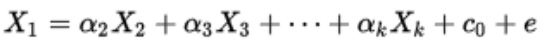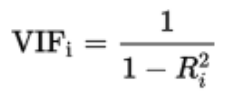## 24. How is Hypothesis testing used in Linear Regression Algorithm?

For the following purposes, we can carry out the Hypothesis testing in linear regression:1. To check whether an independent variable (predictor) is significant or not for the prediction of the target variable. Two common methods for this are —

### By the use of p-values:

If the p-value of a particular independent variable is greater than a certain threshold (usually 0.05), then that independent variable is insignificant for the prediction of the target variable.

### By checking the values of the regression coefficient:

If the value of the regression coefficient corresponding to a particular independent variable is zero, then that variable is insignificant for the predictions of the dependent variable and has no linear relationship with it.

2. To verify whether the calculated regression coefficients i.e, with the help of linear regression algorithm, are good estimators or not of the actual coefficients.

## 25. Is it possible to apply Linear Regression for Time Series Analysis?

Yes, we can apply a linear regression algorithm for doing analysis on time series data, but the results are not promising and hence is not advisable to do so.The reasons behind not preferable linear regression on time-series data are as follows:

• Time series data is mostly used for the prediction of the future but in contrast, linear regression generally seldom gives good results for future prediction as it is basically not meant for extrapolation.
• Moreover, time-series data have a pattern, such as during peak hours, festive seasons, etc., which would most likely be treated as outliers in the linear regression analysis.

## End Notes

I hope you enjoyed the questions and were able to test your knowledge about Linear Regression Algorithm.

If you liked this and want to know more, go visit my other articles on Data Science and Machine Learning by clicking on the Link

Something not mentioned or want to share your thoughts? Feel free to comment below And I’ll get back to you.

## Chirag Goyal

Currently, I am pursuing my Bachelor of Technology (B.Tech) in Computer Science and Engineering from the Indian Institute of Technology Jodhpur(IITJ). I am very enthusiastic about Machine learning, Deep Learning, and Artificial Intelligence.

The media shown in this article on Sign Language Recognition are not owned by Analytics Vidhya and are used at the Author’s discretion.